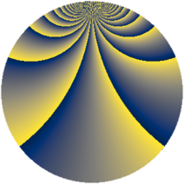# Properties

 Label 2736.2.cnLevel $2736$ Weight $2$ Character orbit 2736.cn Rep. character $\chi_{2736}(113,\cdot)$ Character field $\Q(\zeta_{6})$ Dimension $236$ Sturm bound $960$

# Related objects

## Defining parameters

 Level: $$N$$ $$=$$ $$2736 = 2^{4} \cdot 3^{2} \cdot 19$$ Weight: $$k$$ $$=$$ $$2$$ Character orbit: $$[\chi]$$ $$=$$ 2736.cn (of order $$6$$ and degree $$2$$) Character conductor: $$\operatorname{cond}(\chi)$$ $$=$$ $$171$$ Character field: $$\Q(\zeta_{6})$$ Sturm bound: $$960$$

## Dimensions

The following table gives the dimensions of various subspaces of $$M_{2}(2736, [\chi])$$.

Total New Old
Modular forms 984 244 740
Cusp forms 936 236 700
Eisenstein series 48 8 40

## Trace form

 $$236q - 6q^{5} + 2q^{7} - 8q^{9} + O(q^{10})$$ $$236q - 6q^{5} + 2q^{7} - 8q^{9} + 6q^{11} + 4q^{19} + 6q^{23} + 108q^{25} + 10q^{39} + 14q^{43} - 14q^{45} + 6q^{47} - 108q^{49} + 28q^{55} - 2q^{57} - 2q^{61} - 46q^{63} + 16q^{73} - 6q^{77} + 32q^{81} + 6q^{83} + 8q^{85} - 30q^{87} - 10q^{93} - 36q^{95} + 38q^{99} + O(q^{100})$$

## Decomposition of $$S_{2}^{\mathrm{new}}(2736, [\chi])$$ into newform subspaces

The newforms in this space have not yet been added to the LMFDB.

## Decomposition of $$S_{2}^{\mathrm{old}}(2736, [\chi])$$ into lower level spaces

$$S_{2}^{\mathrm{old}}(2736, [\chi]) \cong$$ $$S_{2}^{\mathrm{new}}(171, [\chi])$$$$^{\oplus 5}$$$$\oplus$$$$S_{2}^{\mathrm{new}}(342, [\chi])$$$$^{\oplus 4}$$$$\oplus$$$$S_{2}^{\mathrm{new}}(684, [\chi])$$$$^{\oplus 3}$$$$\oplus$$$$S_{2}^{\mathrm{new}}(1368, [\chi])$$$$^{\oplus 2}$$